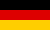# Bri's worldelectronics, programming and more

deutsch### Electronic Calculator

Search two resistors in series or parallel as a more accurate replacement for a single resistor.
Calculates the parts for a second order active lowpass filter.
Calculates the parts for a non-inverting Schmitt Trigger.
Calculates the resistors for a voltage divider for a given voltage output range.
Calculates the resistor for a LM317 voltage regulator.

### Microcontrollers

AVR gcc C/C++ toolchain debian packge and build script.
ARM gcc C/C++ toolchain debian packge and build script for ARM9 and Cortex M4.
GUI for avrdude written in Java for Linux and Windows.
DAC built of discrete resistors with a load at output.
High-end LED fairy lights (each LED can be controlled independently).

### Control theory

Control path step response simulation.
Control path step response simulation with transfer function.
Control loop step response simulation.
Control loop PID controller parameter tuning using iterative algorithm.
PID controller tuning using control path step response to estimate the parameters.
PID controller tuning using stimulated control path oscillation to estimate the parameters. (relay method)
Control path parameter estimation with differential evolution.
Numeric laplace transform inversion for control theory applications.

### Playing around with mathematics

How to draw Mandlebrot and Julia set.

### Primary school excercises

Math practice for addition and subtraction.
Math practice for multiplication.
Math practice for addition and subtraction with large numbers.

### Programming

Free german and english word list.
A tiny Lua IDE and debugger for Linux and Windows.
Some nice java applets. Mostly useless.

### Miscellaneous

My favorite photos.